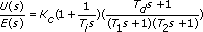# Special Transfer Function

Generates a transfer function model using the PID series algorithm as defined below:where Kc is the proportional gain,

Ti is the integral time in seconds,

and Td is the derivative time in seconds.

T1 is the High frequency rolloff 1 time (in seconds), which defines the time constant of a first order filter in series with the PID algorithm.

T2 is the High frequency rolloff 2 time (in seconds), which defines the time constant of a first order filter in series with the PID algorithm.

ParameterDescription
PID Transfer Function ModelDisplays the mathematical expression that defines the PID transfer function model you create.
Proportional gainRepresents the proportional gain (Kc) of the controller. The default is 1.
Integral time (s)Specifies the controller parameter (Ti) that adjusts the effect of the error integral term on the controller output.
Derivative time (s)Specifies the controller parameter (Td) that adjusts the effect of the error derivative term on the controller output.
High frequency rolloff 1 (s)Defines the cutoff frequency of a low frequency first order filter. If you place a checkmark in the Derivative time (s) checkbox, the step automatically places a checkmark in the High frequency rolloff 1 (s) checkbox.
High frequency rolloff 2 (s)Defines the cutoff frequency of a second low frequency first order filter.